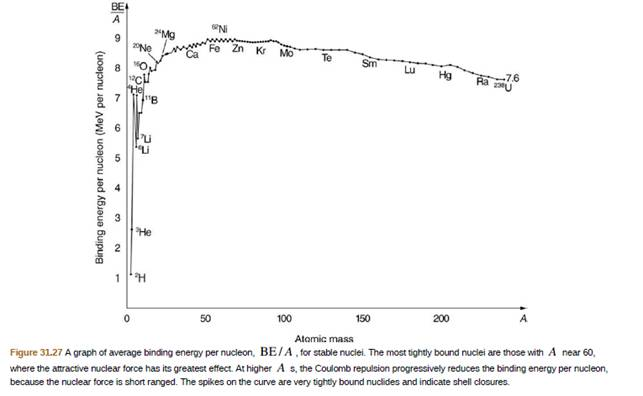Chapter 31, Problem 71PE

Chapter
Section
Textbook Problem

209Bi is the heaviest stable nuclide, and its BE/A is low compared with medium−mass nuclides. Calculate BE/A, the binding energy per nucleon, for 209Bi and compare it with the approximate value obtained from the graph in Figure 31.27.To determine

The binding energy per nucleon of B209 .

Explanation

Given info:

From periodic table,

The atomic mass A of B209 is 209 .

Number of protons Z present in B209 is 83 .

The number of neutron present in N

B209 is N=(AZ)=126 .

Formula used:

Formula to find the B.E. per nucleon is,

B.E.A=Z m p+N m nmtotalc2A

Equation-1

Here, mp is the mass of proton, mn is the mass of neutron, mtotal is the total mass of B209 and c is the speed of light

Still sussing out bartleby?

Check out a sample textbook solution.

See a sample solution

The Solution to Your Study Problems

Bartleby provides explanations to thousands of textbook problems written by our experts, many with advanced degrees!

Get Started